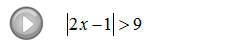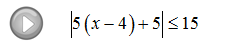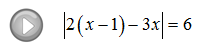Showing posts with label Absolute value. Show all posts
Showing posts with label Absolute value. Show all posts

## Monday, November 12, 2012

### Absolute Value Inequalities

Absolute value inequalities and equations are a bit tricky to work with.  There are basically three cases or situations that can arise when working with them.  By guessing and checking we can answer the following three questions.
Tip: We can easily generalize the above result so that we can use this idea as a template when solving equations and inequalities with absolute values in them. ( Assume n > 0 )

Case 1: | | = n can be solved using X = -n or X = n.
Case 2: | | < n can be solved using -n < X < n.
Case 3: | | > n can be solved using X < -n or X > n.

Use the following steps to solve an absolute value equation or inequality.

Step 1: Isolate the absolute value.
Step 2: Identify the case and apply the appropriate theorem.
Step 3: Solve the resulting equation or inequality.
Step 4: Graph the solution set and express it in interval notation.

Instructional Video: Absolute Value Inequalities

Solve and graph the solution set.
In the three cases listed above notice that we took care to say that n > 0.  The next three problems illustrate some of the situations encountered when n = 0. Plug in some numbers and see what happens.### Absolute Value Equations

Absolute value, by definition, is the distance from zero on a number line. If we have |x| = 3 then the question is, “what number can x be so that the distance to zero is 3?” There are two solutions to this question -3 and 3.
In general,
Here n is a positive integer and X represents an algebraic expression called the argument of the absolute value. To solve these equations, set the argument equal to plus or minus n and solve the resulting equations.

Instructional Video: Absolute Value Equations

Solve.
This technique requires us to first isolate the absolute value. Apply the usual steps for solving to obtain the absolute value alone on one side of the equation, and then set the argument to plus or minus n.
Tip: When in doubt of a solution, check to see if it solves the original equation.  For example, check that {-7, 3} is the solution set for,
Notice that both numbers solve the original equation and therefore we have verified they are in the solution set.